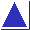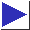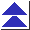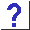# comp.lang.c FAQ list ·Question 20.2

Q: What's a good data structure to use for storing lines of text? I started to use fixed-size arrays of arrays of char, but they're just too restrictive.

A: One good way of doing this is with a pointer (simulating an array) to a set of pointers (each simulating an array) of char. This data structure is sometimes called a ``ragged array,'' and looks something like this:

[FIGURE GOES HERE]

You could set up the tiny array in the figure above with these simple declarations:

```char *a = {"this", "is", "a", "test"};
char **p = a;
```
(where p is the pointer-to-pointer-to-char and a is an intermediate array used to allocate the four pointers-to-char).

To really do dynamic allocation, you'd of course have to call malloc:

```#include <stdlib.h>
char **p = malloc(4 * sizeof(char *));
if(p != NULL) {
p = malloc(5);
p = malloc(3);
p = malloc(2);
p = malloc(5);

if(p && p && p && p) {
strcpy(p, "this");
strcpy(p, "is");
strcpy(p, "a");
strcpy(p, "test");
}
}
```
(Some libraries have a strdup function which would streamline the inner malloc and strcpy calls. It's not Standard, but it's obviously trivial to implement something like it.)

Here is a code fragment which reads an entire file into memory, using the same kind of ragged array. This code is written in terms of the agetline function from question 7.30.

```#include <stdio.h>
#include <stdlib.h>
extern char *agetline(FILE *);
FILE *ifp;

/* assume ifp is open on input file */

char **lines = NULL;
size_t nalloc = 0;
size_t nlines = 0;
char *p;

while((p = agetline(ifp)) != NULL) {
if(nlines >= nalloc) {
nalloc += 50;
#ifdef SAFEREALLOC
lines = realloc(lines, nalloc * sizeof(char *));
#else
if(lines == NULL)		/* in case pre-ANSI realloc */
lines = malloc(nalloc * sizeof(char *));
else	lines = realloc(lines, nalloc * sizeof(char *));
#endif
if(lines == NULL) {
fprintf(stderr, "out of memory");
exit(1);
}
}

lines[nlines++] = p;
}
```
(See the comments on reallocation strategy in question 7.30.)